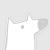Dropout就是指在深度学习网络的训练过程中，对于神经网络单元，按照一定的概率将其暂时从网络中丢弃。Dropout可以减轻过拟合问题。0+0+0+0+0+0+0+0+0+0+0+0+0+0+0+0+0+0+As a result of social network popularity, in recent years, hate speech phenomenon has significantly increased. Due to its harmful effect on minority groups as well as on large communities, there is a pressing need for hate speech detection and filtering. However, automatic approaches shall not jeopardize free speech, so they shall accompany their decisions with explanations and assessment of uncertainty. Thus, there is a need for predictive machine learning models that not only detect hate speech but also help users understand when texts cross the line and become unacceptable. The reliability of predictions is usually not addressed in text classification. We fill this gap by proposing the adaptation of deep neural networks that can efficiently estimate prediction uncertainty. To reliably detect hate speech, we use Monte Carlo dropout regularization, which mimics Bayesian inference within neural networks. We evaluate our approach using different text embedding methods. We visualize the reliability of results with a novel technique that aids in understanding the classification reliability and errors.0+0+0+下载预览0+0+0+0+0+0+0+0+0+0+0+0+0+0+0+0+0+0+0+0+0+Applying Bayesian inference to neural networks often requires approximating the posterior over parameters with simple distributions. The quality of the resulting approximate predictive distribution in function space is poorly understood. We prove that for single hidden layer ReLU networks, there exist simple situations where it is impossible for factorised Gaussian or MC dropout posteriors to give well-calibrated uncertainty estimates. Precisely, they cannot both fit the data confidently and have increased uncertainty in between well-separated clusters of data. This motivates more careful consideration of the consequences of approximate inference in Bayesian neural networks.0+0+0+下载预览
Top